# Logic Introduction to Logic What is logic Logic

• Slides: 74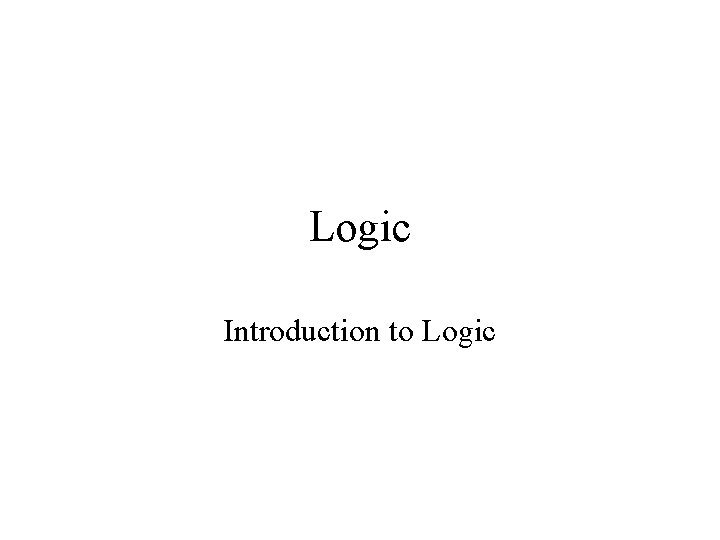Logic Introduction to LogicWhat is logic? • Logic is the study of valid reasoning. • That is, logic tries to establish criteria to decide whether some piece of reasoning is valid or invalid. • OK, so then what do we mean by ‘valid reasoning’?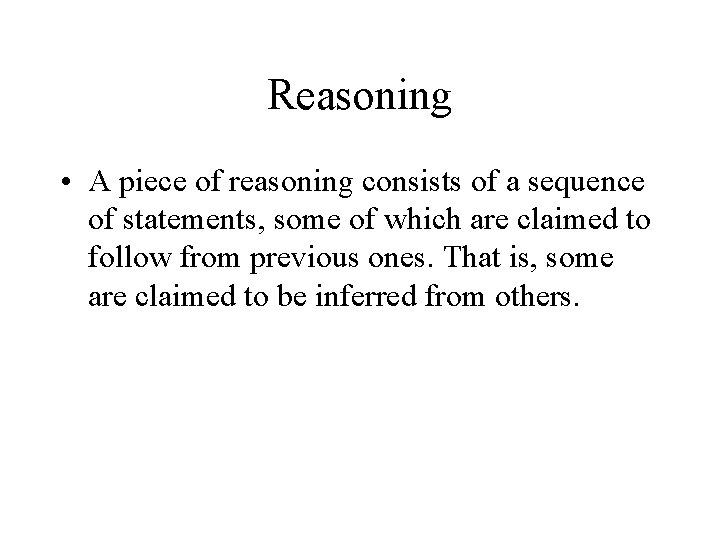Reasoning • A piece of reasoning consists of a sequence of statements, some of which are claimed to follow from previous ones. That is, some are claimed to be inferred from others.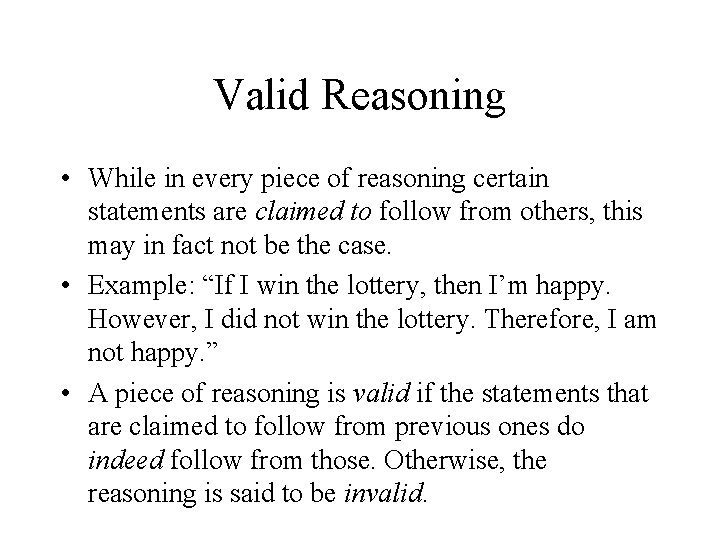Valid Reasoning • While in every piece of reasoning certain statements are claimed to follow from others, this may in fact not be the case. • Example: “If I win the lottery, then I’m happy. However, I did not win the lottery. Therefore, I am not happy. ” • A piece of reasoning is valid if the statements that are claimed to follow from previous ones do indeed follow from those. Otherwise, the reasoning is said to be invalid.Sound Reasoning • Not all valid reasoning is good reasoning. • Example: “If I win the lottery, then I’ll be poor. So, since I did win the lottery, I am poor. ” • This piece of reasoning is valid, but not very good, since it assumed an illogical claim (‘If I win the lottery, I’ll be poor. ’ Huh? ? ) • Sound reasoning is valid reasoning based on acceptable assumptions.Truth and Implication • Logic studies the validity of reasoning. • Logic does not study soundness. • Therefore, logic alone cannot tell us whether an argument is good. Hence, logic alone is not a guide to truth. • Instead, logic can tell us, assuming certain things to be true, what else will be true as well. Thus, logic is a guide to implication.Arguments, Premises and Conclusion • In logic, pieces of reasoning are analyzed using the notion of an argument • An argument consists of any number of premises, and one conclusion • Again, in logic, we are merely interested in whether the conclusion follows from the premises: we are not interested in whether those premises are true or acceptable.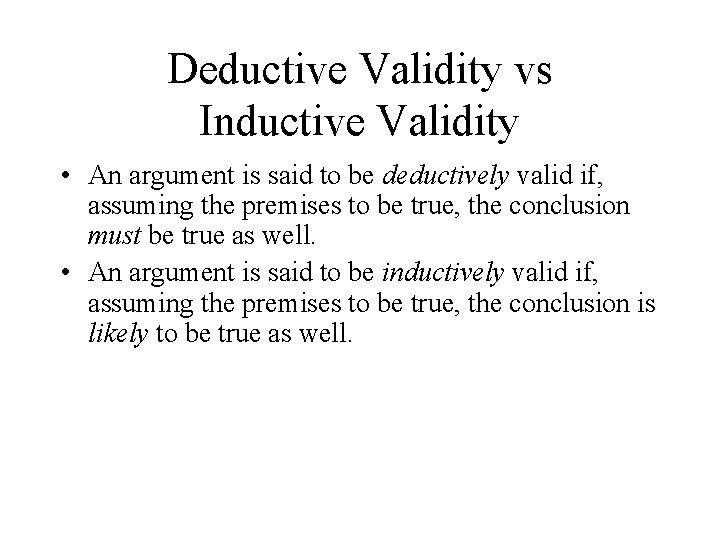Deductive Validity vs Inductive Validity • An argument is said to be deductively valid if, assuming the premises to be true, the conclusion must be true as well. • An argument is said to be inductively valid if, assuming the premises to be true, the conclusion is likely to be true as well.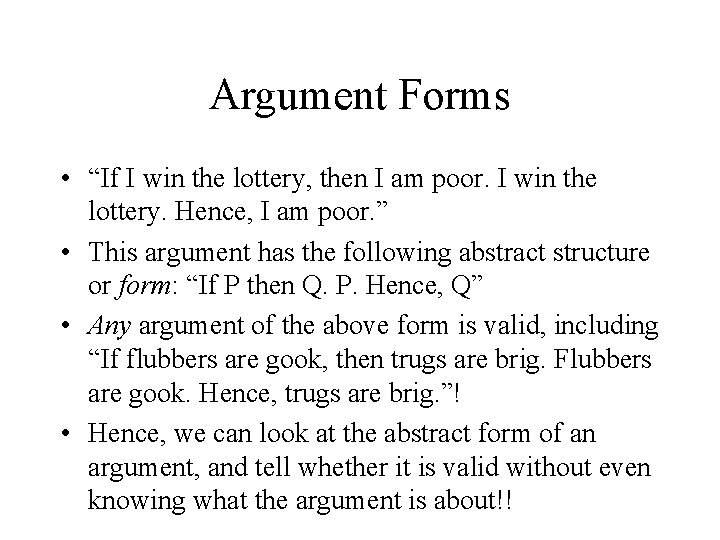Argument Forms • “If I win the lottery, then I am poor. I win the lottery. Hence, I am poor. ” • This argument has the following abstract structure or form: “If P then Q. P. Hence, Q” • Any argument of the above form is valid, including “If flubbers are gook, then trugs are brig. Flubbers are gook. Hence, trugs are brig. ”! • Hence, we can look at the abstract form of an argument, and tell whether it is valid without even knowing what the argument is about!!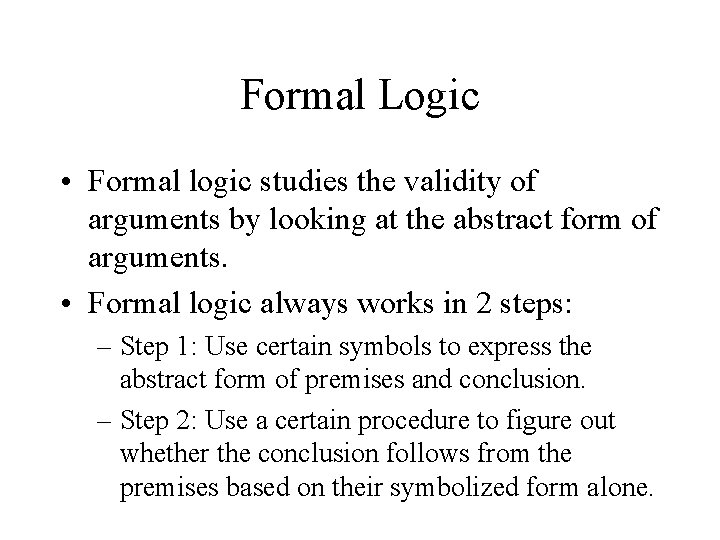Formal Logic • Formal logic studies the validity of arguments by looking at the abstract form of arguments. • Formal logic always works in 2 steps: – Step 1: Use certain symbols to express the abstract form of premises and conclusion. – Step 2: Use a certain procedure to figure out whether the conclusion follows from the premises based on their symbolized form alone.Propositional Logic • Propositional Logic studies validity at the level of simple and compound propositions. • Simple proposition: An expression that has a truth value (a claim or a statement). E. g. “Ali is tall” • Compound proposition: An expression that combines simple propositions using truthfunctional connectives like ‘and’, ‘or’, ‘not’, and ‘if … then’. E. g. “Ali is tall and M is smart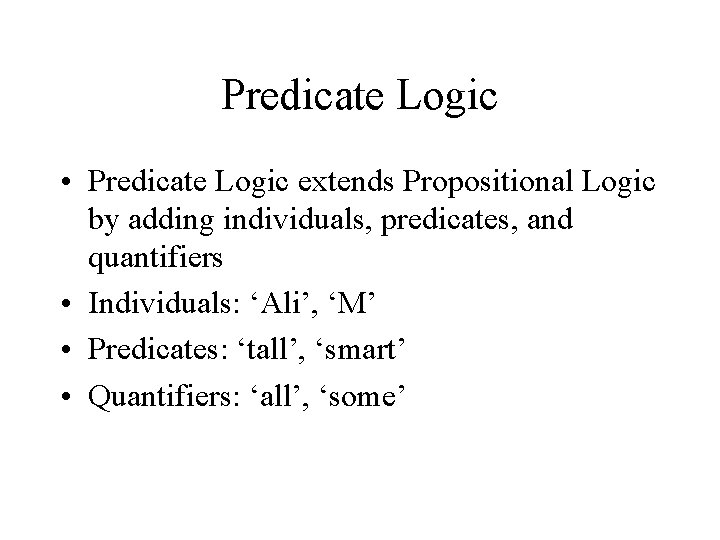Predicate Logic • Predicate Logic extends Propositional Logic by adding individuals, predicates, and quantifiers • Individuals: ‘Ali’, ‘M’ • Predicates: ‘tall’, ‘smart’ • Quantifiers: ‘all’, ‘some’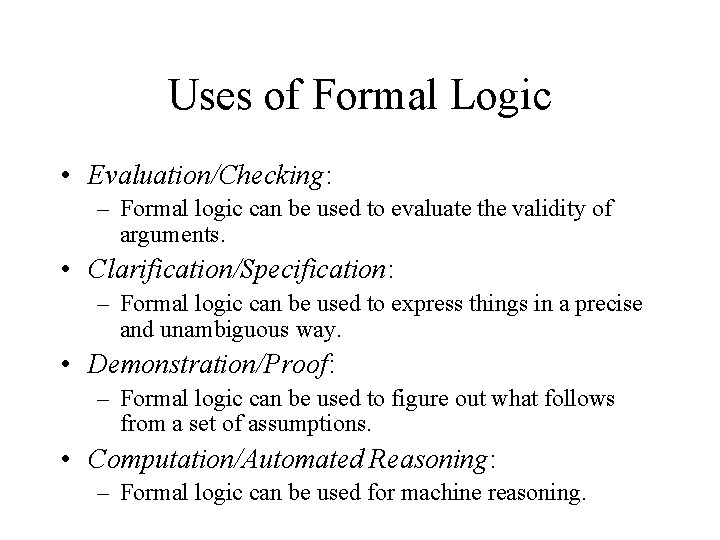Uses of Formal Logic • Evaluation/Checking: – Formal logic can be used to evaluate the validity of arguments. • Clarification/Specification: – Formal logic can be used to express things in a precise and unambiguous way. • Demonstration/Proof: – Formal logic can be used to figure out what follows from a set of assumptions. • Computation/Automated Reasoning: – Formal logic can be used for machine reasoning.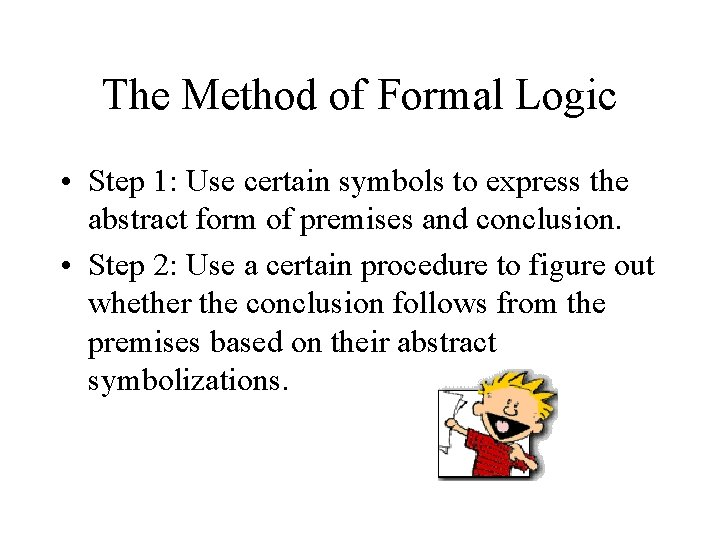The Method of Formal Logic • Step 1: Use certain symbols to express the abstract form of premises and conclusion. • Step 2: Use a certain procedure to figure out whether the conclusion follows from the premises based on their abstract symbolizations.FOL • FOL (the language of First-Order Logic): The formal language that we use to symbolize statements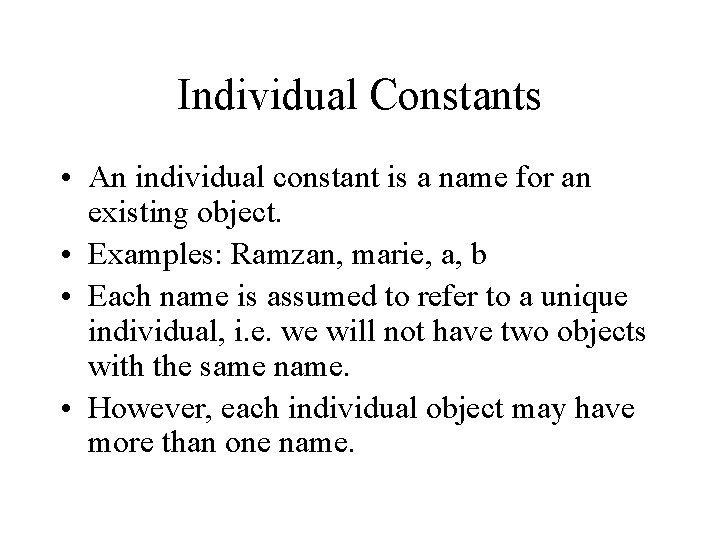Individual Constants • An individual constant is a name for an existing object. • Examples: Ramzan, marie, a, b • Each name is assumed to refer to a unique individual, i. e. we will not have two objects with the same name. • However, each individual object may have more than one name.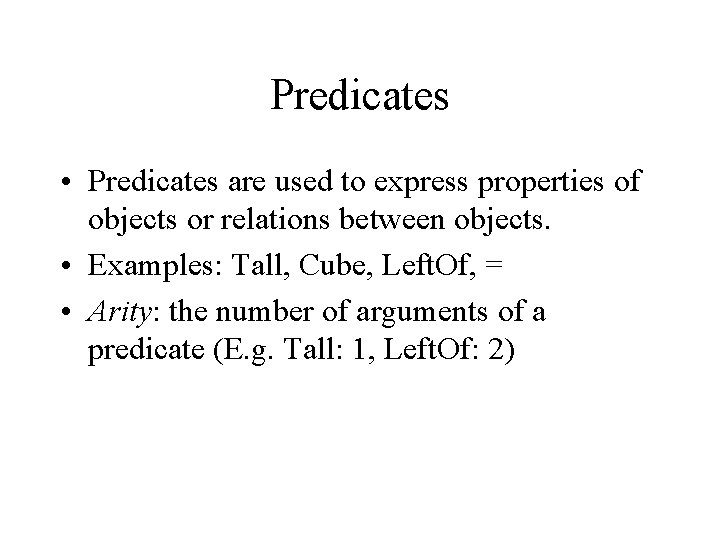Predicates • Predicates are used to express properties of objects or relations between objects. • Examples: Tall, Cube, Left. Of, = • Arity: the number of arguments of a predicate (E. g. Tall: 1, Left. Of: 2)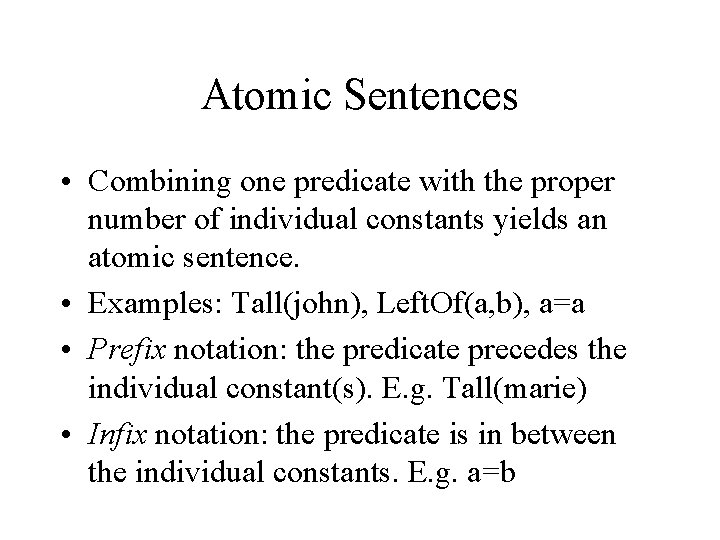Atomic Sentences • Combining one predicate with the proper number of individual constants yields an atomic sentence. • Examples: Tall(john), Left. Of(a, b), a=a • Prefix notation: the predicate precedes the individual constant(s). E. g. Tall(marie) • Infix notation: the predicate is in between the individual constants. E. g. a=bArgument, Premises, Conclusion, Validity and Soundness • An argument consists of 0 or more premises and 1 conclusion. • An argument is (deductively) valid iff: – assuming its premises are true, its conclusion must be true as well. • An argument is (deductively) sound iff: – it is valid, and – its premises are true. • In pure logic, we are only interested in validity.Demonstrating Invalidity • An argument is valid if it is impossible for the conclusion to be false while the premises are true. • Thus, to demonstrate invalidity, all we have to do is to demonstrate that it is possible for the conclusion to be false while the premises are true. • The easiest way to do this is to come up with a scenario (or possible world) in which all premises are true and the conclusion false.Demonstrating Validity • To demonstrate validity, we have to show that there is no possible way for all premises to be true and the conclusion false all at the same time. • Showing a scenario in which all premises are true, and in which the conclusion is true as well, does not demonstrate validity, b/c there may still be a different scenario in which all premises are true and the conclusion false. • Obviously, this holds true in general. Hence, showing one possible scenario cannot demonstrate validity! • Of course, we could try and generate all relevant possible worlds, but this method is either impractical (there are too many), or simply impossible (there are infinitely many).Step-by-step Reasoning • OK, so what do we do? Well, we can do what we do in everyday reasoning: we start with the premises, and we gradually work our way to the conclusion: “Either the housemaid or the butler killed Mr. X. Now, we know that if the housemaid would have done it, the alarm would have gone off. But, the alarm did not go off. Therefore, the housemaid did not do it. So, since it was either the housemaid or the butler, it must have been the butler. ”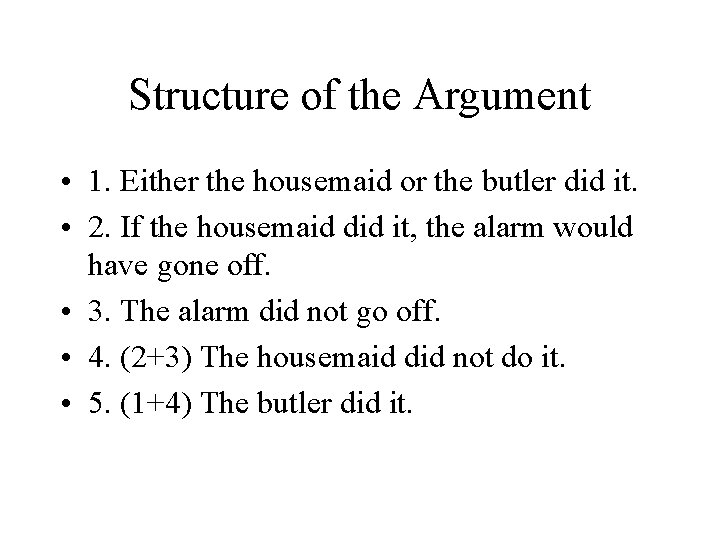Structure of the Argument • 1. Either the housemaid or the butler did it. • 2. If the housemaid did it, the alarm would have gone off. • 3. The alarm did not go off. • 4. (2+3) The housemaid did not do it. • 5. (1+4) The butler did it.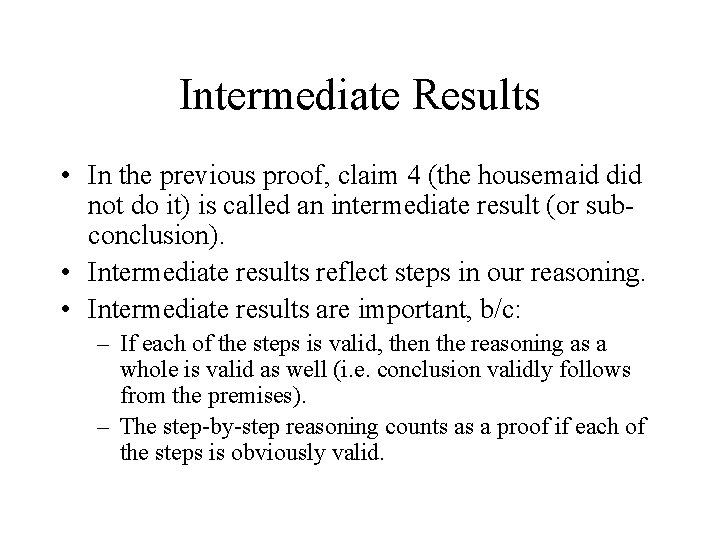Intermediate Results • In the previous proof, claim 4 (the housemaid did not do it) is called an intermediate result (or subconclusion). • Intermediate results reflect steps in our reasoning. • Intermediate results are important, b/c: – If each of the steps is valid, then the reasoning as a whole is valid as well (i. e. conclusion validly follows from the premises). – The step-by-step reasoning counts as a proof if each of the steps is obviously valid.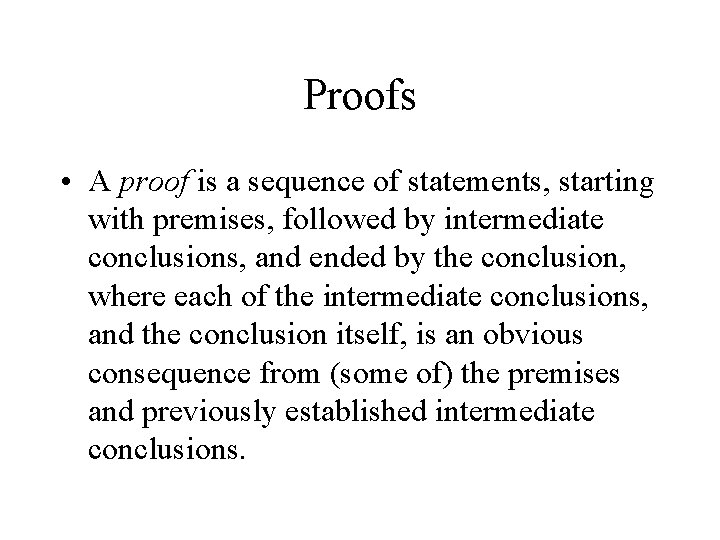Proofs • A proof is a sequence of statements, starting with premises, followed by intermediate conclusions, and ended by the conclusion, where each of the intermediate conclusions, and the conclusion itself, is an obvious consequence from (some of) the premises and previously established intermediate conclusions.Formal Proofs • Formal proofs try to formalize proofs by: – Symbolizing the statements in a proof (again, we will use FOL for this) – Spelling out what we count as an ‘obvious consequence’ based on this symbolizationWhat is ‘obvious’ is not obvious • Problem: ‘obvious’ is a bit of a vague term, as what is obvious to some, may not be obvious to others. So, what are going to count as ‘obvious’? • We are going to play it safe: In formal proofs, we are only going to allow steps that are about as obvious as we can get. Thus, we are only going to allow ‘baby inferences’. • In formal proofs, bigger inferences, which may still be obvious to many (if not all of us), will still have to be broken up into smaller ones!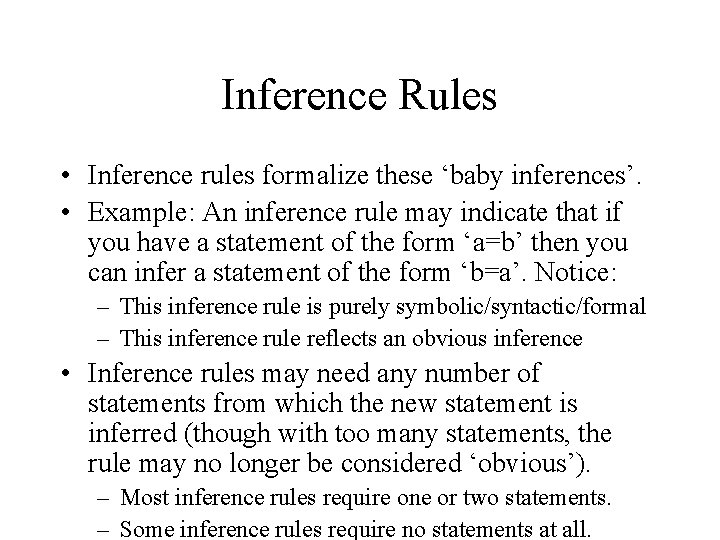Inference Rules • Inference rules formalize these ‘baby inferences’. • Example: An inference rule may indicate that if you have a statement of the form ‘a=b’ then you can infer a statement of the form ‘b=a’. Notice: – This inference rule is purely symbolic/syntactic/formal – This inference rule reflects an obvious inference • Inference rules may need any number of statements from which the new statement is inferred (though with too many statements, the rule may no longer be considered ‘obvious’). – Most inference rules require one or two statements. – Some inference rules require no statements at all.Formal Systems • There are many formal systems of logic, each with their own predefined set of inference rules: – First of all, the nature of the inference rules depends on the symbols that the system uses to express statements. – Moreover, even if two systems use the same symbols, they may still have different inference rules.Connectives: From Atomic Claims to Complex Claims • So far, we have only seen atomic claims: claims consisting of a single predicate. E. g. “a is to the right of b”. • Boolean connectives: and, or, not, if … then, if and only if • We can combine atomic claims using boolean connectives to form complex claims. E. g. “Either a is to the right of b or a is to the left of b”.Propositional Logic • Propositional Logic is the logic involving complex claims as constructed from atomic claims and boolean connectives.Truth-Functional Connectives • Boolean Connectives are usually called truth-functional connectives. • The truth value of a complex claim that has been constructed using a truth-functional connective is a function of the truth value of the claims that are being connected by that connective.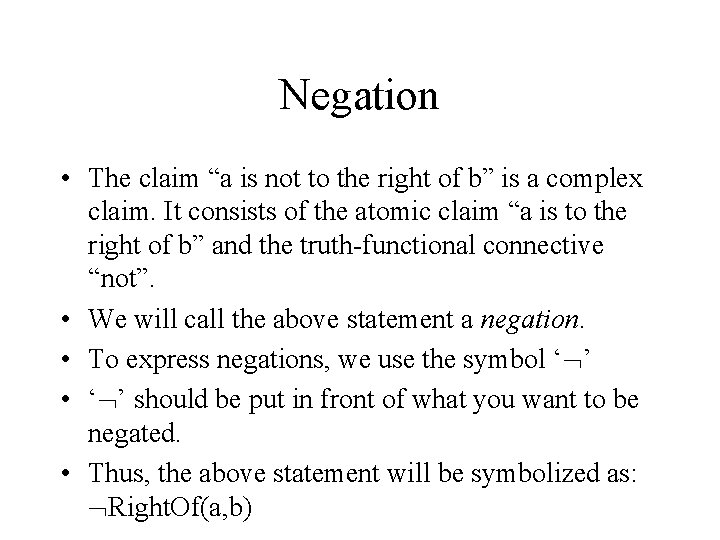Negation • The claim “a is not to the right of b” is a complex claim. It consists of the atomic claim “a is to the right of b” and the truth-functional connective “not”. • We will call the above statement a negation. • To express negations, we use the symbol ‘ ’ • ‘ ’ should be put in front of what you want to be negated. • Thus, the above statement will be symbolized as: Right. Of(a, b)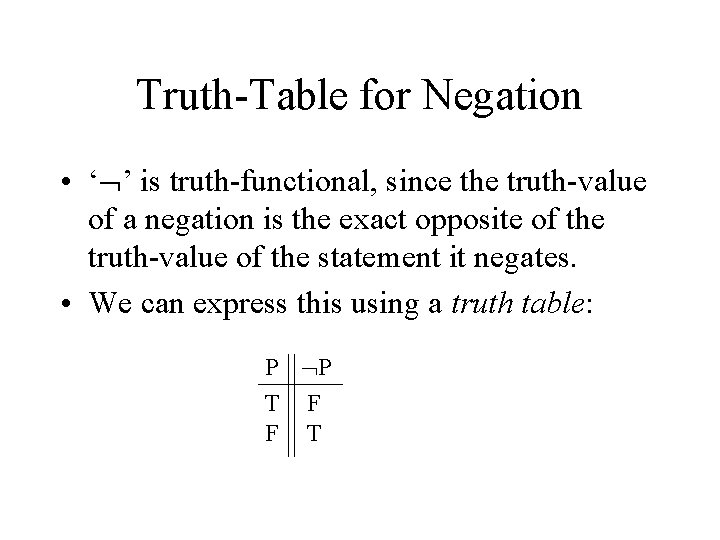Truth-Table for Negation • ‘ ’ is truth-functional, since the truth-value of a negation is the exact opposite of the truth-value of the statement it negates. • We can express this using a truth table: P P T F F T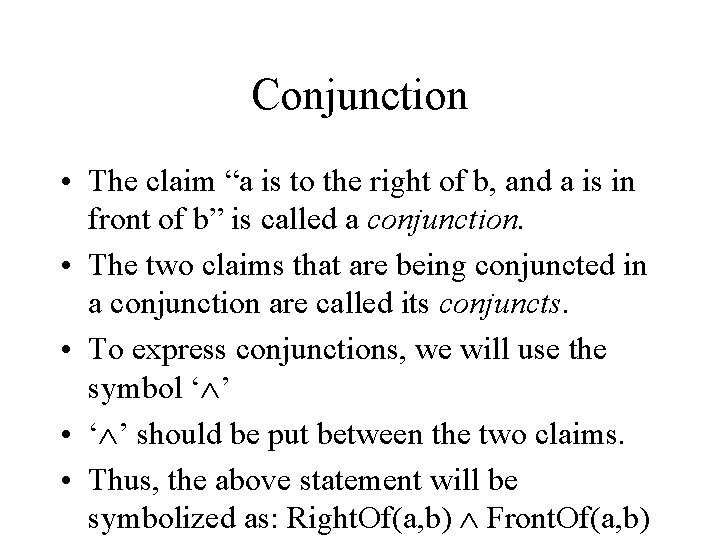Conjunction • The claim “a is to the right of b, and a is in front of b” is called a conjunction. • The two claims that are being conjuncted in a conjunction are called its conjuncts. • To express conjunctions, we will use the symbol ‘ ’ • ‘ ’ should be put between the two claims. • Thus, the above statement will be symbolized as: Right. Of(a, b) Front. Of(a, b)Truth-Table for Conjunction • ‘ ’ is truth-functional, since a conjunction is true when both conjuncts are true, and it is false otherwise. • Again, we can show this using a truth table: P T T F F Q P Q T T F F F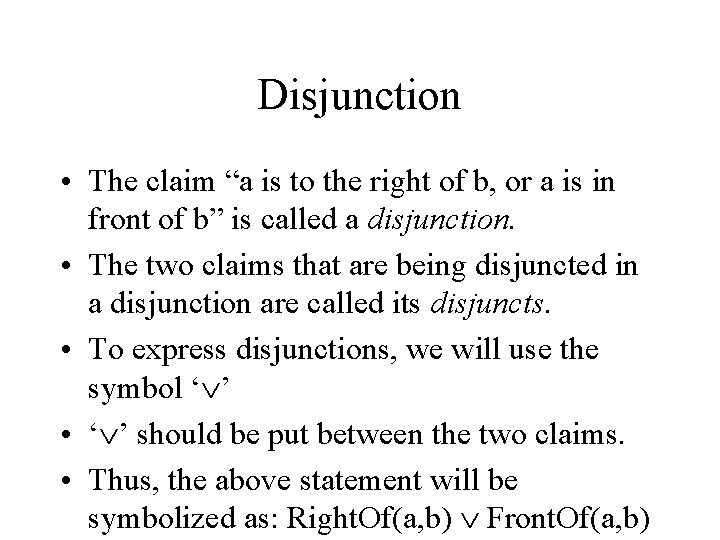Disjunction • The claim “a is to the right of b, or a is in front of b” is called a disjunction. • The two claims that are being disjuncted in a disjunction are called its disjuncts. • To express disjunctions, we will use the symbol ‘ ’ • ‘ ’ should be put between the two claims. • Thus, the above statement will be symbolized as: Right. Of(a, b) Front. Of(a, b)Truth-Table for Disjunction • ‘ ’ is truth-functional, since a disjunction is true when at least one of its disjuncts is true, and it is false otherwise. • Again, we can show this using a truth table: P T T F F Q P Q T T F T T T F F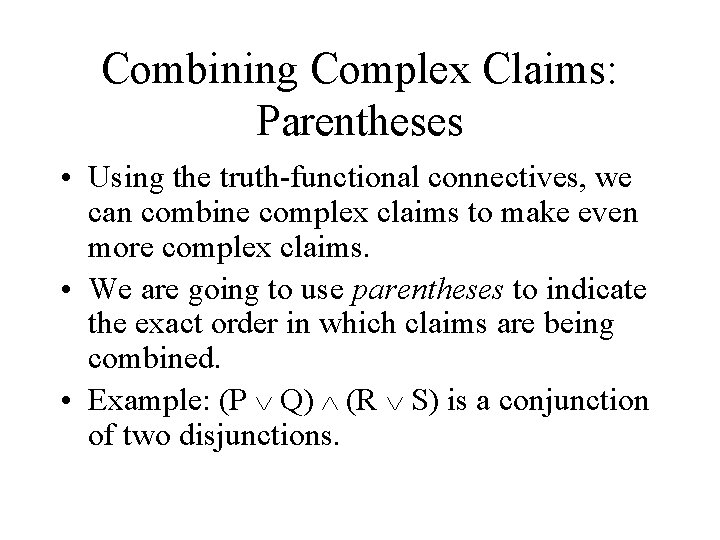Combining Complex Claims: Parentheses • Using the truth-functional connectives, we can combine complex claims to make even more complex claims. • We are going to use parentheses to indicate the exact order in which claims are being combined. • Example: (P Q) (R S) is a conjunction of two disjunctions.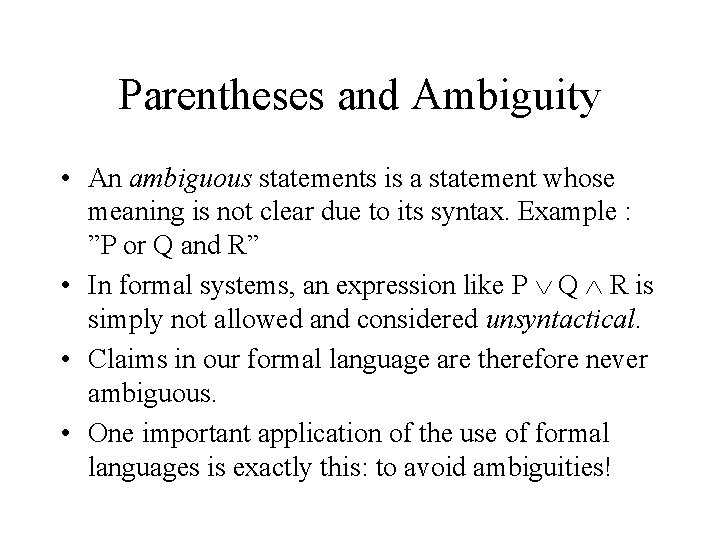Parentheses and Ambiguity • An ambiguous statements is a statement whose meaning is not clear due to its syntax. Example : ”P or Q and R” • In formal systems, an expression like P Q R is simply not allowed and considered unsyntactical. • Claims in our formal language are therefore never ambiguous. • One important application of the use of formal languages is exactly this: to avoid ambiguities!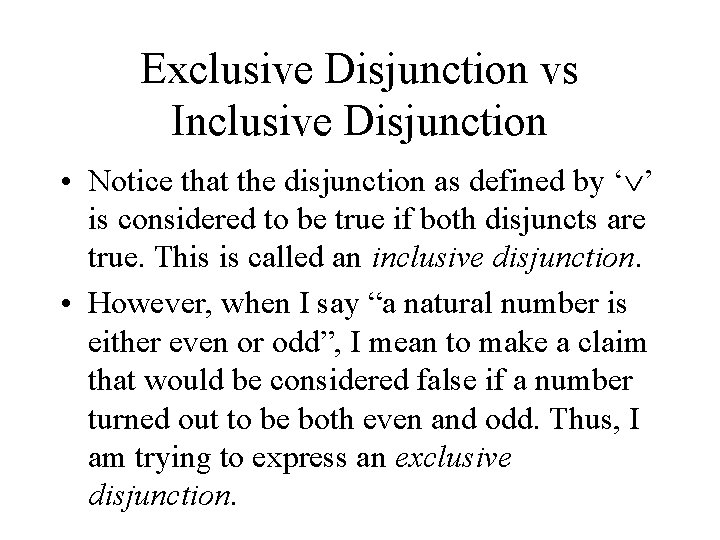Exclusive Disjunction vs Inclusive Disjunction • Notice that the disjunction as defined by ‘ ’ is considered to be true if both disjuncts are true. This is called an inclusive disjunction. • However, when I say “a natural number is either even or odd”, I mean to make a claim that would be considered false if a number turned out to be both even and odd. Thus, I am trying to express an exclusive disjunction.How to express Exclusive Disjunctions • We could define a separate symbol for exclusive disjunctions, but we are not going to do that. • Fortunately, exclusive disjunctions can be expressed using the symbols we already have: (P Q) P T T F F Q (P Q) (P Q) T T F F T T T F F T F !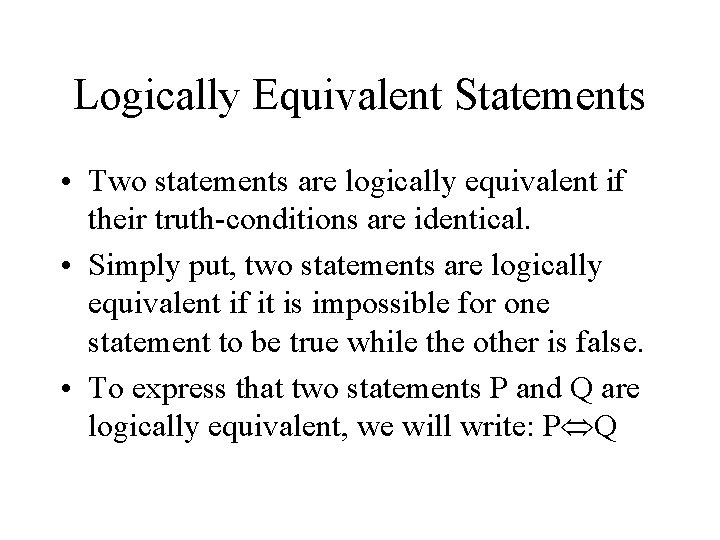Logically Equivalent Statements • Two statements are logically equivalent if their truth-conditions are identical. • Simply put, two statements are logically equivalent if it is impossible for one statement to be true while the other is false. • To express that two statements P and Q are logically equivalent, we will write: P QSome Important Equivalences • Double Negation: – P P • De. Morgan: – – (P Q) P Q • Distribution: – – P (Q R) (P Q) (P R)Some Other Equivalences • Commutation: –P Q Q P • Association: – P (Q R) (P Q) R • Idempotence: –P P PTautologies • A tautology is a statement that is necessarily true. • Example: P P • Any statement that evaluates to True in every row of its truth-table is a tautology.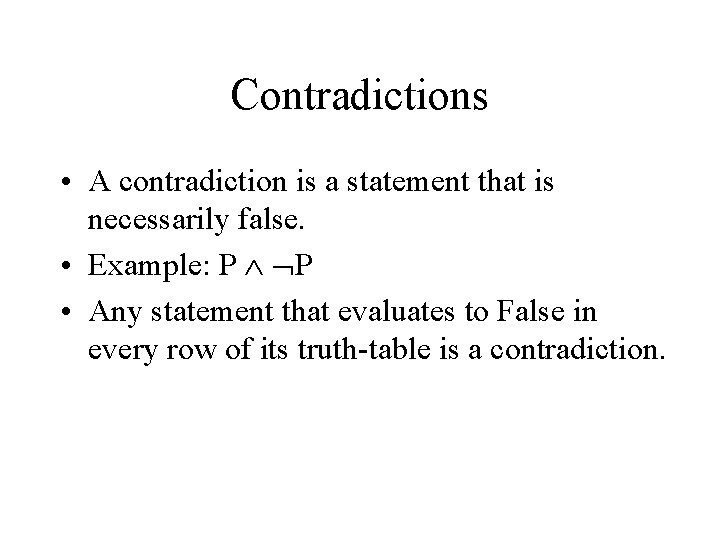Contradictions • A contradiction is a statement that is necessarily false. • Example: P P • Any statement that evaluates to False in every row of its truth-table is a contradiction.Equivalences • Two statements are equivalent if they have the exact same truth-conditions. • Example: P and P • In every row of their combined truth-table, two equivalent statements are either both true or both false.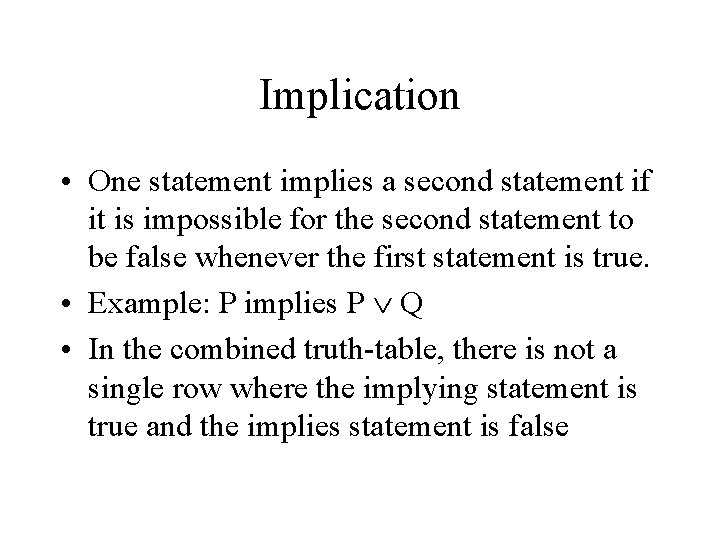Implication • One statement implies a second statement if it is impossible for the second statement to be false whenever the first statement is true. • Example: P implies P Q • In the combined truth-table, there is not a single row where the implying statement is true and the implies statement is falseConsistency • A set of statements is consistent if it is possible for all of them to be true at the same time. • Example: {P, P Q, Q} • In the combined truth-table of a consistent set of statements there is at least one row where they all evaluate to True.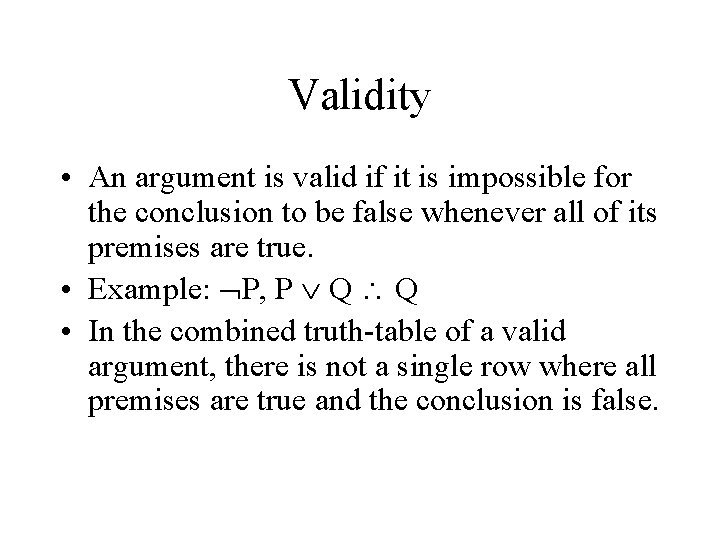Validity • An argument is valid if it is impossible for the conclusion to be false whenever all of its premises are true. • Example: P, P Q Q • In the combined truth-table of a valid argument, there is not a single row where all premises are true and the conclusion is false.The Principle of Substitution of Logical Equivalents • Let us write S(P) for a sentence which has P as a component part, and let us write S(Q) for the result of substituting Q for P in S(P). • The principle of substitution of logical equivalents states that if P Q, then S(P) S(Q). • Example: – Since Small(a), it is also true that (Cube(a) Small(a)) (Cube(a) Small(a))Simplifying Statements • Using the principle of substitution of logical equivalents, and using the logical equivalences that we saw before (Double Negation, Association, Commutation, Idempotence, De. Morgan, and Distibution), we can often simplify statements. • Example: (A B) A (Commutation) (B A) A (Association) B (A A) (Idempotence) B ANegation Normal Form • Literals: Atomic Sentences or negations thereof. • Negation Normal Form: An expression built up with ‘ ’, and literals. • Using repeated De. Morgan and Double Negation, we can transform any truth-functional expression built up with ‘ ’, and ‘ ’ into an expression that is in Negation Normal Form. • Example: ((A B) C) (De. Morgan) (A B) C (Double Neg, De. M) ( A B) C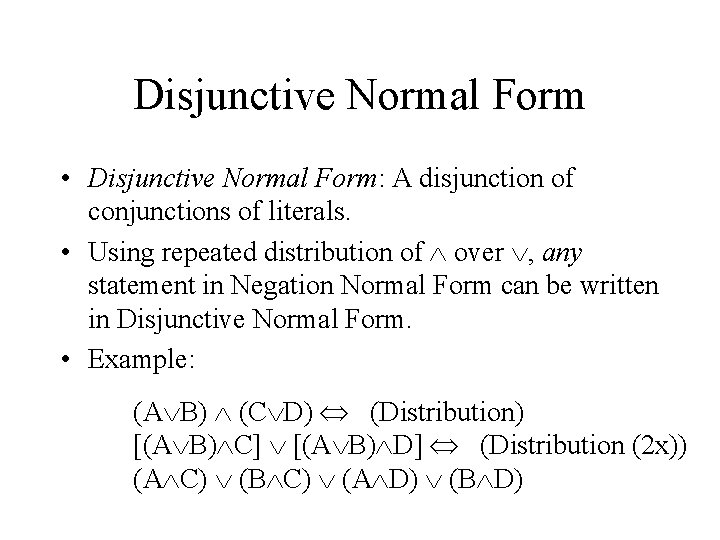Disjunctive Normal Form • Disjunctive Normal Form: A disjunction of conjunctions of literals. • Using repeated distribution of over , any statement in Negation Normal Form can be written in Disjunctive Normal Form. • Example: (A B) (C D) (Distribution) [(A B) C] [(A B) D] (Distribution (2 x)) (A C) (B C) (A D) (B D)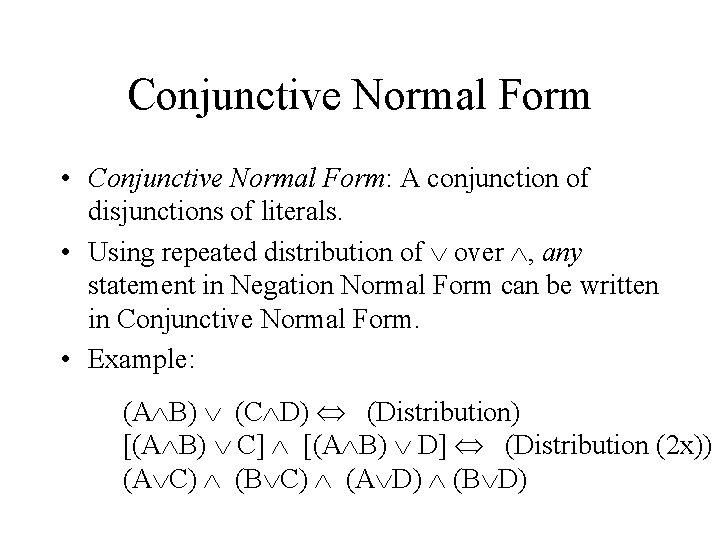Conjunctive Normal Form • Conjunctive Normal Form: A conjunction of disjunctions of literals. • Using repeated distribution of over , any statement in Negation Normal Form can be written in Conjunctive Normal Form. • Example: (A B) (C D) (Distribution) [(A B) C] [(A B) D] (Distribution (2 x)) (A C) (B C) (A D) (B D)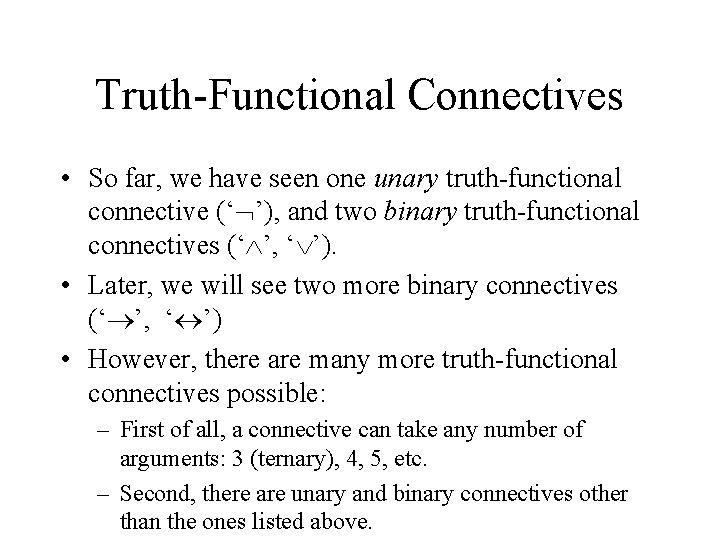Truth-Functional Connectives • So far, we have seen one unary truth-functional connective (‘ ’), and two binary truth-functional connectives (‘ ’, ‘ ’). • Later, we will see two more binary connectives (‘ ’, ‘ ’) • However, there are many more truth-functional connectives possible: – First of all, a connective can take any number of arguments: 3 (ternary), 4, 5, etc. – Second, there are unary and binary connectives other than the ones listed above.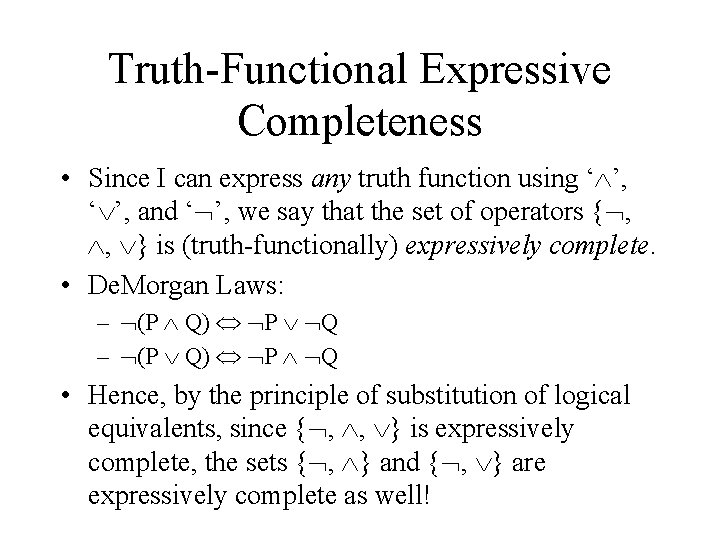Truth-Functional Expressive Completeness • Since I can express any truth function using ‘ ’, and ‘ ’, we say that the set of operators { , , } is (truth-functionally) expressively complete. • De. Morgan Laws: – (P Q) P Q • Hence, by the principle of substitution of logical equivalents, since { , , } is expressively complete, the sets { , } and { , } are expressively complete as well!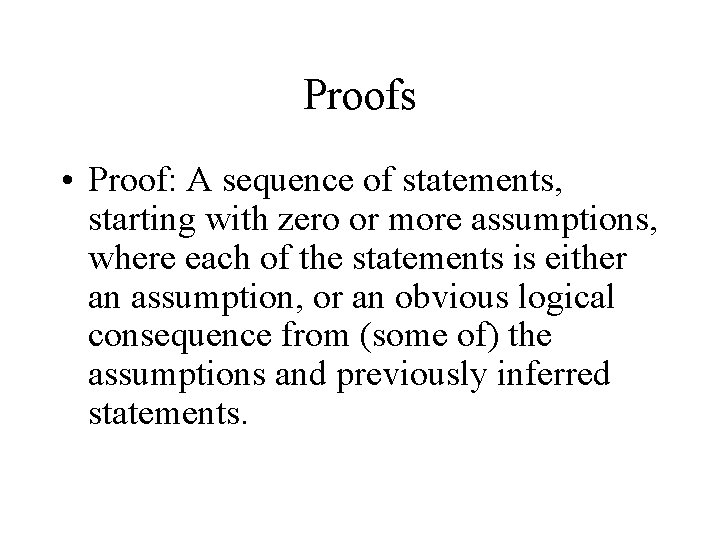Proofs • Proof: A sequence of statements, starting with zero or more assumptions, where each of the statements is either an assumption, or an obvious logical consequence from (some of) the assumptions and previously inferred statements.Proof by Contradiction • ‘Assuming P to be the case, then I get some kind of impossibility or contradiction. Hence, contrary to my assumption, P cannot be the case. ’ • This pattern of reasoning is called Proof by Contradiction (or Indirect Proof or Reductio ad Absurdum or simply Reductio).‘ ’ • The symbol ‘ ’ is used to express a logical contradiction. • Theorem: A statement is a logical contradiction iff • Theorem: A set of statements is logically inconsistent iff { 1, …, n}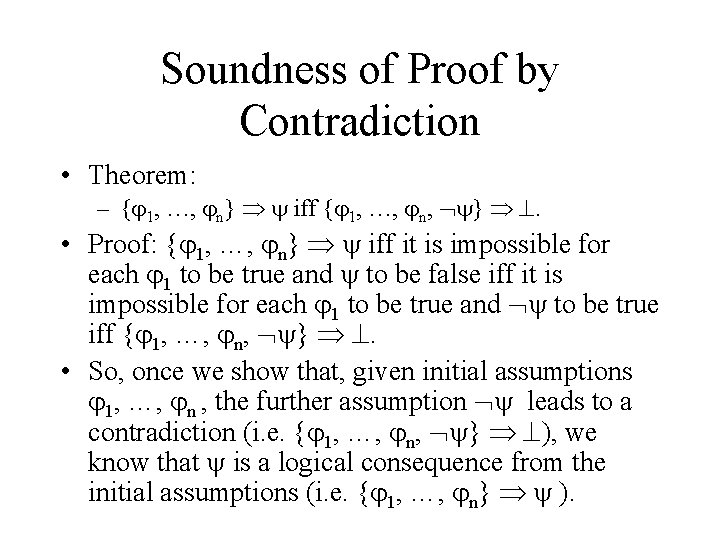Soundness of Proof by Contradiction • Theorem: – { 1, …, n} iff { 1, …, n, } . • Proof: { 1, …, n} iff it is impossible for each 1 to be true and to be false iff it is impossible for each 1 to be true and to be true iff { 1, …, n, } . • So, once we show that, given initial assumptions 1, …, n , the further assumption leads to a contradiction (i. e. { 1, …, n, } ), we know that is a logical consequence from the initial assumptions (i. e. { 1, …, n} ).The Practice and Art of Formal Proofs • Given that formal proofs are not mechanical, and thus require some amount of creativity, the only way to learn to do formal proofs is through practice, and more practice! • Giving formal proofs is a skill and an art. In fact, you will probably develop your own style in making proofs.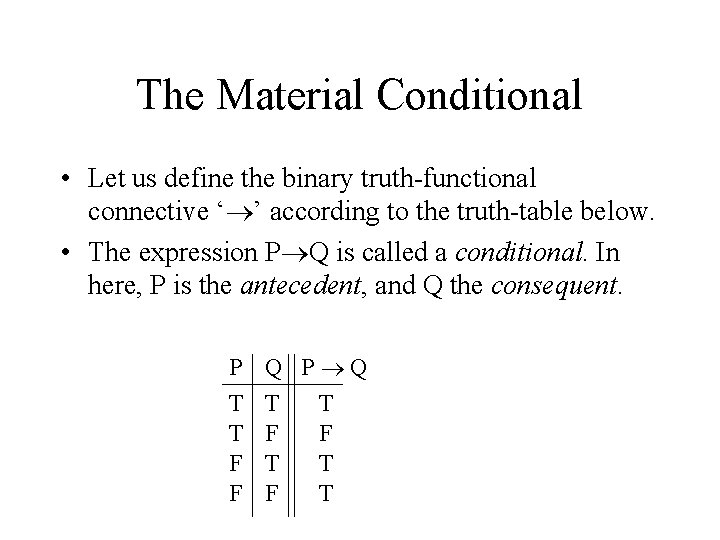The Material Conditional • Let us define the binary truth-functional connective ‘ ’ according to the truth-table below. • The expression P Q is called a conditional. In here, P is the antecedent, and Q the consequent. P T T F F Q P Q T T F F T T F T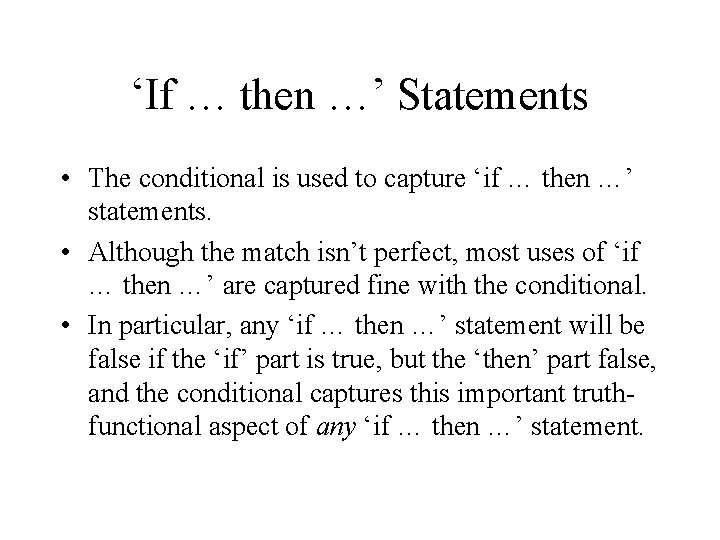‘If … then …’ Statements • The conditional is used to capture ‘if … then …’ statements. • Although the match isn’t perfect, most uses of ‘if … then …’ are captured fine with the conditional. • In particular, any ‘if … then …’ statement will be false if the ‘if’ part is true, but the ‘then’ part false, and the conditional captures this important truthfunctional aspect of any ‘if … then …’ statement.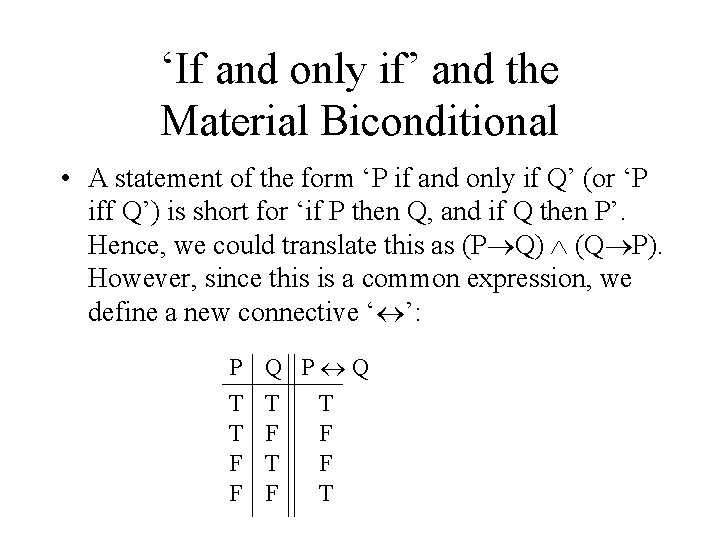‘If and only if’ and the Material Biconditional • A statement of the form ‘P if and only if Q’ (or ‘P iff Q’) is short for ‘if P then Q, and if Q then P’. Hence, we could translate this as (P Q) (Q P). However, since this is a common expression, we define a new connective ‘ ’: P T T F F Q P Q T T F F T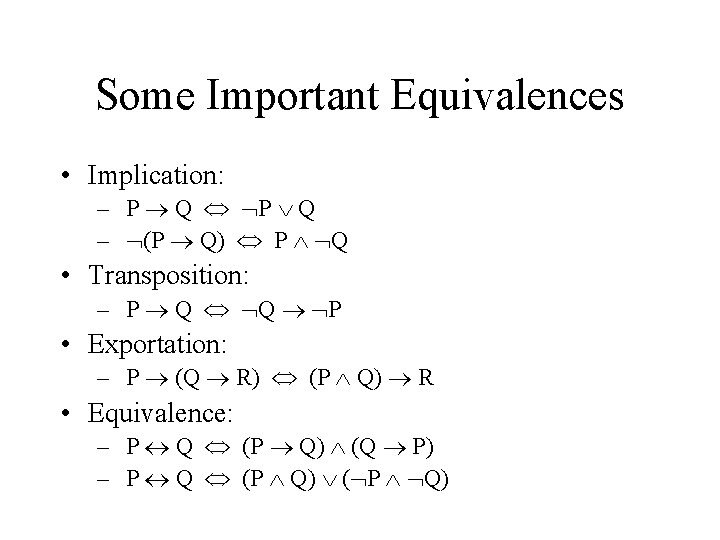Some Important Equivalences • Implication: – P Q – (P Q) P Q • Transposition: – P Q Q P • Exportation: – P (Q R) (P Q) R • Equivalence: – P Q (P Q) (Q P) – P Q (P Q) ( P Q)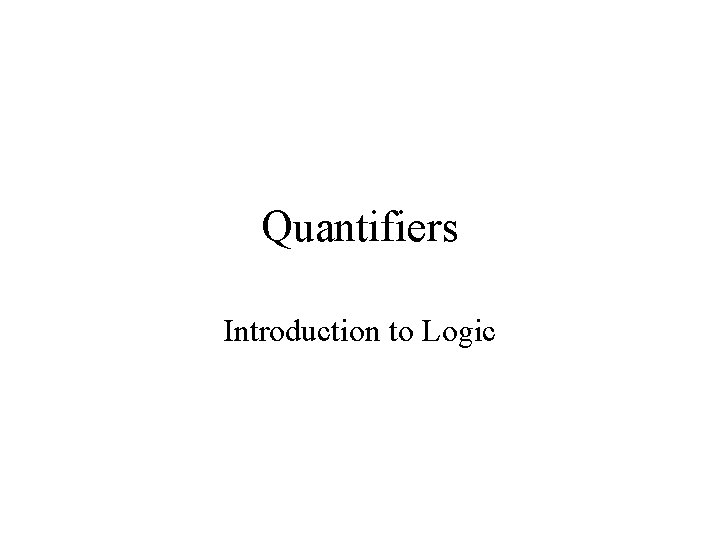Quantifiers Introduction to Logic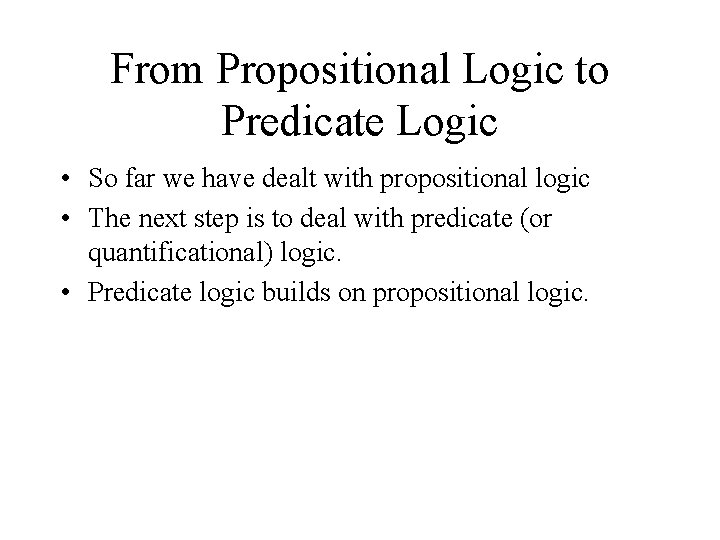From Propositional Logic to Predicate Logic • So far we have dealt with propositional logic • The next step is to deal with predicate (or quantificational) logic. • Predicate logic builds on propositional logic.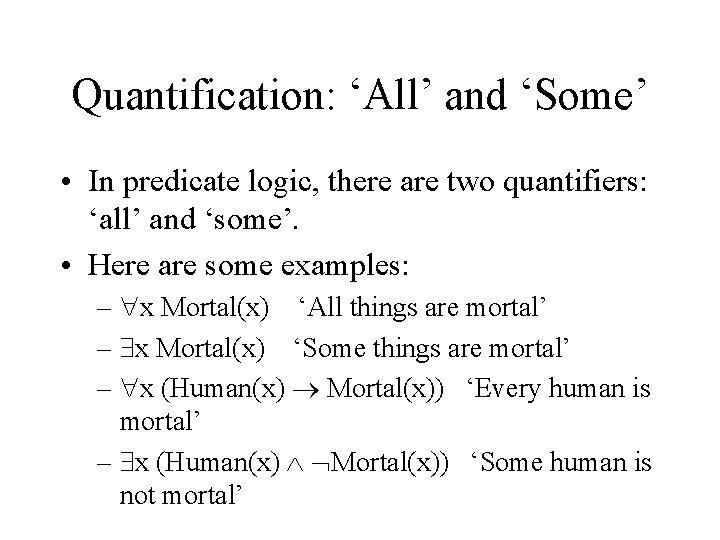Quantification: ‘All’ and ‘Some’ • In predicate logic, there are two quantifiers: ‘all’ and ‘some’. • Here are some examples: – x Mortal(x) ‘All things are mortal’ – x Mortal(x) ‘Some things are mortal’ – x (Human(x) Mortal(x)) ‘Every human is mortal’ – x (Human(x) Mortal(x)) ‘Some human is not mortal’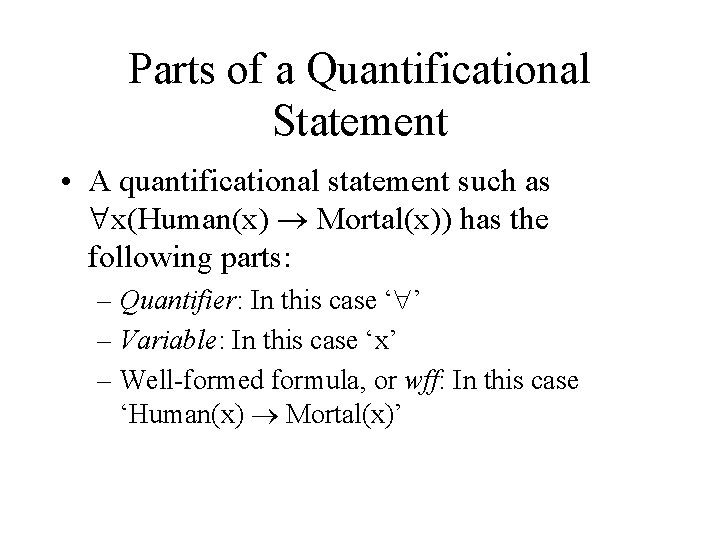Parts of a Quantificational Statement • A quantificational statement such as x(Human(x) Mortal(x)) has the following parts: – Quantifier: In this case ‘ ’ – Variable: In this case ‘x’ – Well-formed formula, or wff: In this case ‘Human(x) Mortal(x)’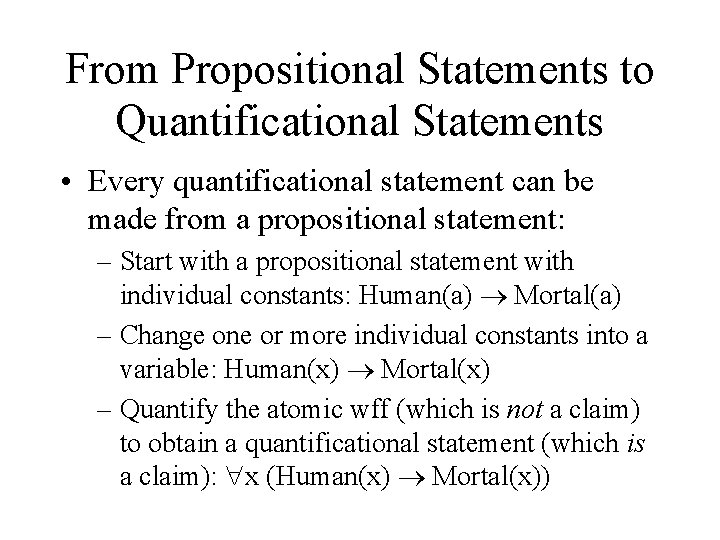From Propositional Statements to Quantificational Statements • Every quantificational statement can be made from a propositional statement: – Start with a propositional statement with individual constants: Human(a) Mortal(a) – Change one or more individual constants into a variable: Human(x) Mortal(x) – Quantify the atomic wff (which is not a claim) to obtain a quantificational statement (which is a claim): x (Human(x) Mortal(x))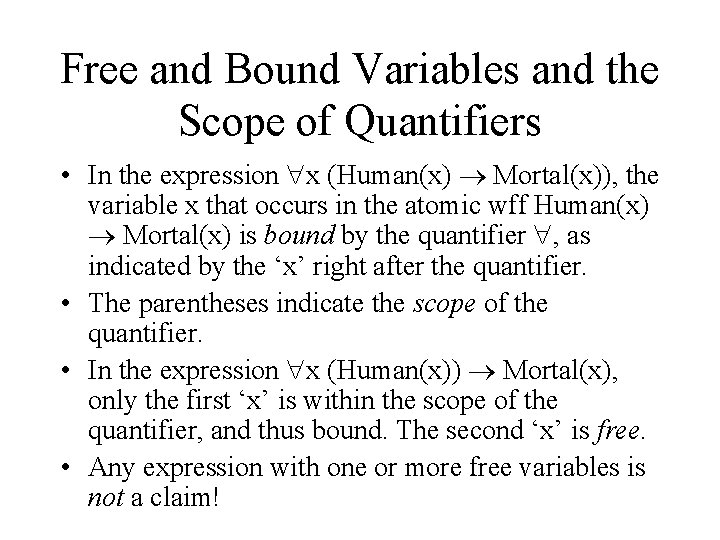Free and Bound Variables and the Scope of Quantifiers • In the expression x (Human(x) Mortal(x)), the variable x that occurs in the atomic wff Human(x) Mortal(x) is bound by the quantifier , as indicated by the ‘x’ right after the quantifier. • The parentheses indicate the scope of the quantifier. • In the expression x (Human(x)) Mortal(x), only the first ‘x’ is within the scope of the quantifier, and thus bound. The second ‘x’ is free. • Any expression with one or more free variables is not a claim!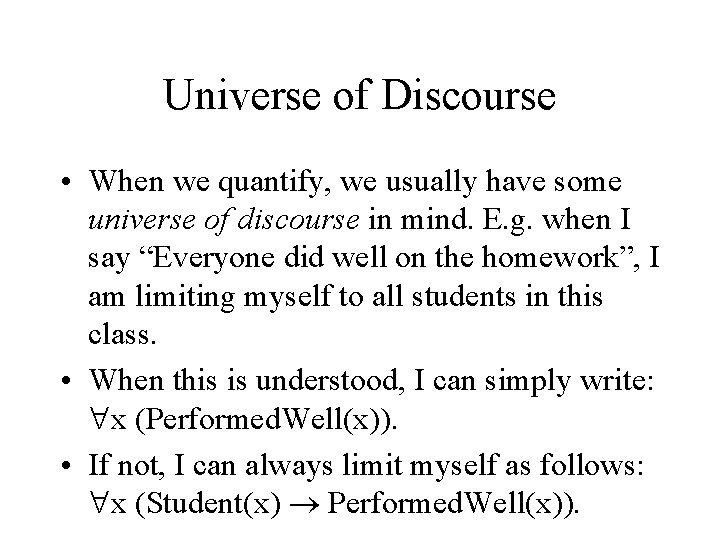Universe of Discourse • When we quantify, we usually have some universe of discourse in mind. E. g. when I say “Everyone did well on the homework”, I am limiting myself to all students in this class. • When this is understood, I can simply write: x (Performed. Well(x)). • If not, I can always limit myself as follows: x (Student(x) Performed. Well(x)).